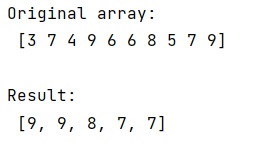# Fast way to find the n-largest values of an array using NumPy

Learn, how to find the n-largest values of an array using NumPy in Python?
Submitted by Pranit Sharma, on February 14, 2023

NumPy is an abbreviated form of Numerical Python. It is used for different types of scientific operations in python. Numpy is a vast library in python which is used for almost every kind of scientific or mathematical operation. It is itself an array which is a collection of various methods and functions for processing the arrays.

## Finding the n-largest values of a NumPy array

Suppose that we are given a NumPy array that contains integer values and we need to find the largest N values in this numpy array.

We will use the heapq module for this purpose, it provides the implementation for heap queue algorithm in python. It would be easy for us to find the N largest element in a NumPy array.

The heapq module provides nlargest() method which takes values N and the array for which we need to find the largest values.

Let us understand with the help of an example,

## Python code to find the n-largest values of an array using NumPy

```# Import numpy
import numpy as np

# Importing heapq
import heapq

# Creating a numpy array
arr = np.array([3,7,4,9,6,6,8,5,7,9])

# Display original array
print("Original array:\n",arr,"\n")

# Finding 5 largest values
res = heapq.nlargest(5,arr)

# Display result
print("Result:\n",res)
```

Output: